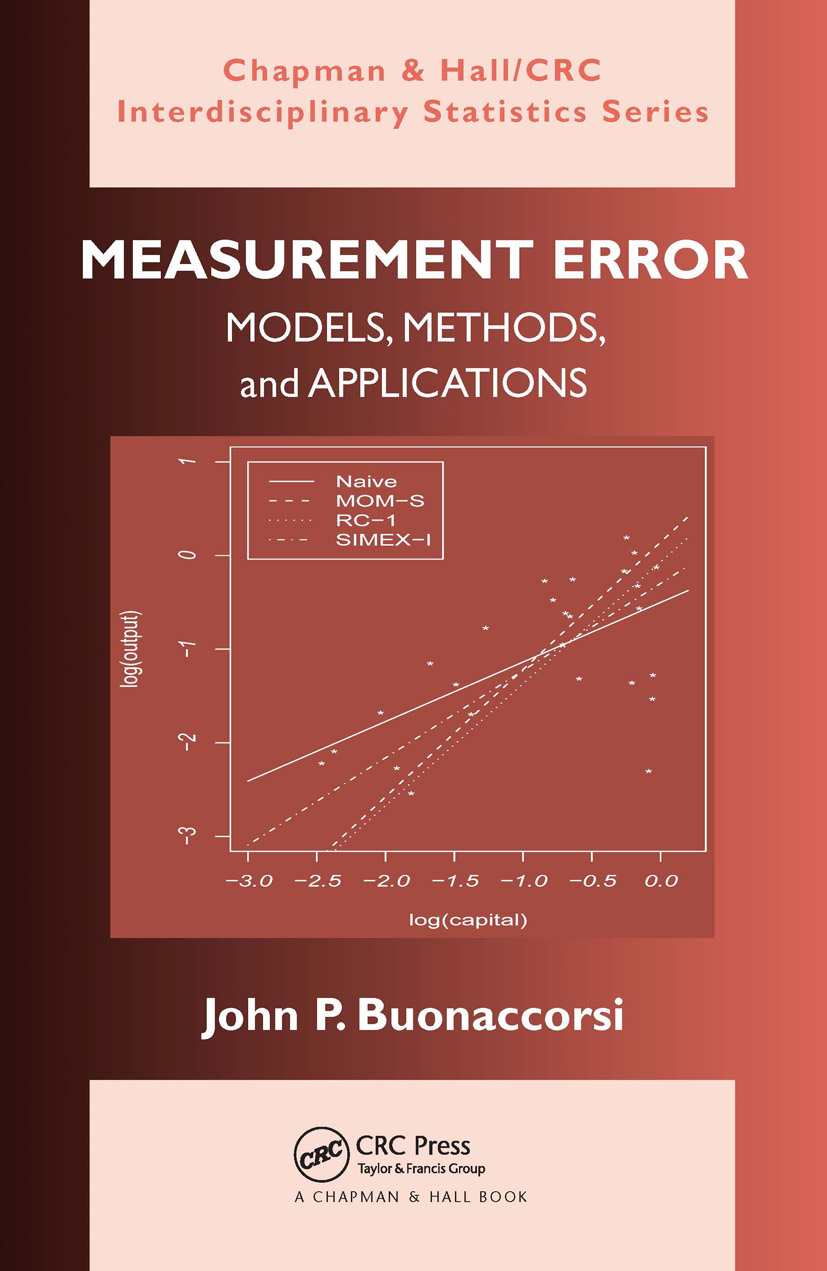# Measurement Error

## Models, Methods, and Applications, 1st Edition

Chapman and Hall/CRC

464 pages | 30 B/W Illus.

Hardback: 9781420066562
pub: 2010-03-02
SAVE ~\$25.00
\$125.00
\$100.00
x
eBook (VitalSource) : 9780429150357
pub: 2010-03-02
from \$28.98

FREE Standard Shipping!

### Description

Over the last 20 years, comprehensive strategies for treating measurement error in complex models and accounting for the use of extra data to estimate measurement error parameters have emerged. Focusing on both established and novel approaches, Measurement Error: Models, Methods, and Applications provides an overview of the main techniques and illustrates their application in various models. It describes the impacts of measurement errors on naive analyses that ignore them and presents ways to correct for them across a variety of statistical models, from simple one-sample problems to regression models to more complex mixed and time series models.

The book covers correction methods based on known measurement error parameters, replication, internal or external validation data, and, for some models, instrumental variables. It emphasizes the use of several relatively simple methods, moment corrections, regression calibration, simulation extrapolation (SIMEX), modified estimating equation methods, and likelihood techniques. The author uses SAS-IML and Stata to implement many of the techniques in the examples.

Accessible to a broad audience, this book explains how to model measurement error, the effects of ignoring it, and how to correct for it. More applied than most books on measurement error, it describes basic models and methods, their uses in a range of application areas, and the associated terminology.

### Reviews

The author has written a praiseworthy summary of available results on measurement errors in a wide variety of statistical models. The author also covers results described in very recent papers, which have not been previously published in any other book. … The book brings a big help for theoretical researchers as well as applied statisticians who deal with data contaminated by measurement errors. The author demonstrates a very deep understanding for the theory and does not hesitate to discuss many specific theoretical problems. He succeeds very well in illustrating the methods on real examples and explaining the ideas to applied statisticians. Although not primarily intended for biostatisticians, I would say the book is suitable exactly for epidemiological and biostatistical applications. … very clearly and systematically organized. … the book offers an excellent and remarkable overview of available methods for incorporating measurement errors to statistical analysis.

—Jan Kalina, ISCB News, 52, December 2011

… we think Buonaccorsi’s book would be a great textbook … The book also contains many interesting data examples, which are useful for those concerned with applications. Overall, the book is also a good reference resource … We would recommend this book to people who are interested in statistical methods for measurement error.

—C.Y. Wang and X. Song, The American Statistician, August 2011

This book is a successful attempt to collect, organize and present the literature over the newly developed and earlier existing topics of measurement error models in one place. …The material that is presented in chapters [11 and 12] is, to my knowledge, not available in any other book on this area. … This book should be of immense help to those who are interested in the theoretical as well as applied aspects of measurement error models. … Some topics in the book may be used to teach advanced graduate courses. … The book is overall well written, presents updated developments in the area of measurement error models and is an excellent guide to applications. I am sure that it will stimulate researchers in and newcomers to this area.

Journal of the Royal Statistical Society, Series A, April 2011

There are plenty of illustrations and worked examples throughout … The book is very readable and clearly demonstrates the importance of recognizing measurement error, which is often ignored as a bit of a nuisance to be swept under the carpet. Together with easily accessible software, in the future, the problem is likely to be more commonly addressed and dealt with properly.

International Statistical Review (2010), 78, 3

Introduction

What is measurement error?

Some examples

The main ingredients

Some terminology

Misclassification in Estimating a Proportion

Motivating examples

A model for the true values

Misclassification models and naive analyses

Correcting for misclassification

Finite populations

Multiple measures with no direct validation

The multinomial case

Mathematical developments

Misclassification in Two-Way Tables

Introduction

Models for true values

Misclassification models and naive estimators

Behavior of naive analyses

Correcting using external validation data

Correcting using internal validation data

General two-way tables

Mathematical developments

Simple Linear Regression

Introduction

The additive Berkson model and consequences

The behavior of naive analyses

Examples

Residual analysis

Prediction

Mathematical developments

Multiple Linear Regression

Introduction

Model for true values

Models and bias in naive estimators

Correcting for measurement error

Weighted and other estimators

Examples

Instrumental variables

Mathematical developments

Measurement Error in Regression: A General Overview

Introduction

Models for true values

Analyses without measurement error

Measurement error models

Extra data

Assessing bias in naive estimators

Assessing bias using induced models

Assessing bias via estimating equations

Moment based and direct bias corrections

Regression calibration and quasi-likelihood methods

Simulation extrapolation (SIMEX)

Correcting using likelihood methods

Modified estimating equation approaches

Correcting for misclassification

Overview on use of validation data

Bootstrapping

Mathematical developments

Binary Regression

Introduction

Using validation data

Misclassification of predictors

Introduction

First-order models with interaction

General nonlinear functions of the predictors

Linear measurement error with validation data

Misclassification of a categorical predictor

Miscellaneous

Nonlinear Regression

Poisson regression: Cigarettes and cancer rates

General nonlinear models

Error in the Response

Introduction

Additive error in a single sample

Linear measurement error in the one-way setting

Measurement error in the response in linear models

Mixed/Longitudinal Models

Introduction, overview, and some examples

Berkson error in designed repeated measures

Additive error in the linear mixed model

Time Series

Introduction

Random walk/population viability models

Linear autoregressive models

Background Material

Notation for vectors, covariance matrices, etc.

Double expectations

Approximate Wald inferences

The delta-method: approximate moments of nonlinear functions

Fieller’s method for ratios

References

Author Index

Subject Index